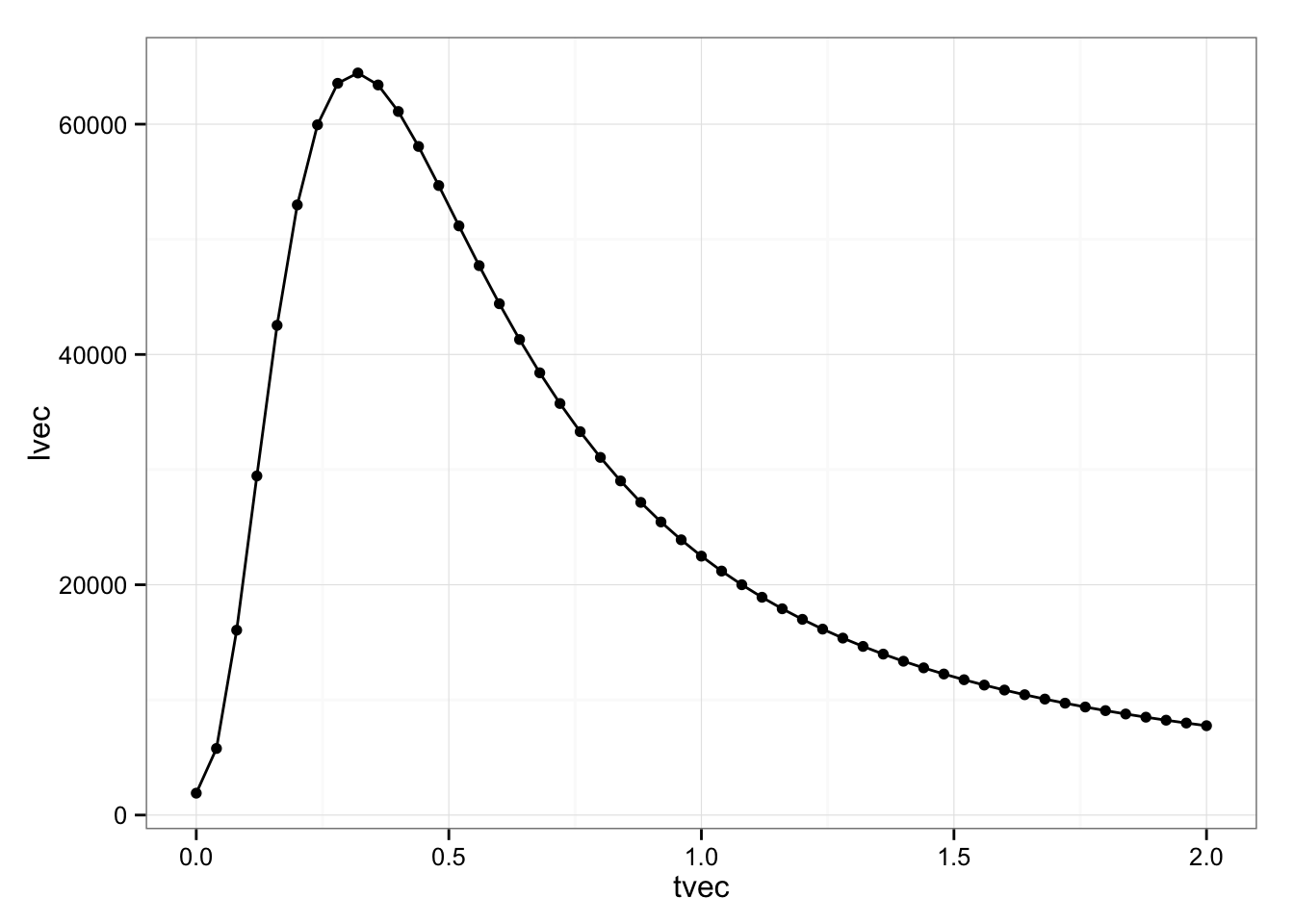Someone asked whether it was possible to fit a mixed model in lme4 with box constraints on the fixed-effect parameters. It is, although (1) it requires a little bit of extra hacking (see below) and (2) it works most easily for generalized (non-Gaussian) linear MMs rather than LMMs (but see the second section).

library("lme4")
library("ggplot2"); theme_set(theme_bw())

Fit the basic (unconstrained) model:

gm0 <- glmer(cbind(incidence, size - incidence) ~ period + (1 | herd),
data = cbpp, family = binomial)
(beta0 <- fixef(gm0))
## (Intercept)     period2     period3     period4
##   -1.398343   -0.991925   -1.128216   -1.579745

Start following the sequence laid out in ?modular:

glmod <- glFormula(cbind(incidence, size - incidence) ~ period + (1 | herd),
data = cbpp, family = binomial)
devfun <- do.call(mkGlmerDevfun, glmod)

glmer uses a two-stage optimization process. During the first stage (nAGQ=0) we can’t constrain the fixed effect parameters (they’re profiled out of the optimization), so we just run optimizeGlmer in the usual way from ?modular:

opt1 <- optimizeGlmer(devfun)

Now set up the next optimization:

theta.lwr <- environment(devfun)$lower ## this changes after update! devfun <- updateGlmerDevfun(devfun, glmod$reTrms)
rho <- environment(devfun)
nbeta <- ncol(rho$pp$X)
theta <- rho$pp$theta

Now do the second-stage optimization, by hand rather than using optimizeGlmer(...,stage=2) as in ?modular. The code below is actually a little bit simpler than the guts of optimizeGlmer, because it’s not handling as wide a range of cases (e.g. user-specified starting values, additional control parameters, …). Instead of the usual constraints (theta parameters bounded below by rho$lower, which corresponds to 0 for diagonal and -Inf for off-diagonal elements, fixed-effect parameters unbounded), we constrain the fixed-effects parameters other than the intercept to [-1,1]. opt2 <- nloptwrap(par=c(rho$pp$theta,rep(0,nbeta)), fn=devfun, lower=c(theta.lwr,-Inf,rep(-1,nbeta-1)), upper=c(rep(Inf,length(theta)),Inf,rep(1,nbeta-1))) opt2$par
##   0.6682799 -1.5037866 -0.8798190 -1.0000000 -1.0000000
opt2$fval ##  186.114 ## compare with 2*log(L) of original model -2*c(logLik(gm0)) ##  184.0531 Now we have the estimated parameters and log-likelihood, but it’s a little bit prettier if we put the results into a merMod object. (The only place we take a shortcut below is in the mc argument, for which we’ll just substitute the matched call from the original model. This will only be a problem if we try to use update() on the fitted model …) (gm1 <- mkMerMod(rho, opt2, glmod$reTrms, glmod$fr, mc=gm0@call)) ## Generalized linear mixed model fit by maximum likelihood (Laplace ## Approximation) [glmerMod] ## Family: binomial ( logit ) ## Formula: cbind(incidence, size - incidence) ~ period + (1 | herd) ## Data: cbpp ## AIC BIC logLik deviance df.resid ## 196.1140 206.2408 -93.0570 186.1140 51 ## Random effects: ## Groups Name Std.Dev. ## herd (Intercept) 0.6683 ## Number of obs: 56, groups: herd, 15 ## Fixed Effects: ## (Intercept) period2 period3 period4 ## -1.5038 -0.8798 -1.0000 -1.0000 Alternatively, it is (at least in principle) possible to skip the nAGQ=0 stage entirely and just fit the final model; this might be a good idea if the reason for constraining the fixed-effect parameters was to keep them away from a bad region. The stuff below here is not really working properly. You probably shouldn’t even bother reading on unless you’re interested in the guts of lme4. ## For linear mixed models This is harder for LMMs because by default lmer profiles out the fixed effect parameters, so we have to work a little bit harder to run a GLMM with a Gaussian response. All of the latter part of the machinery stays the same, so we’ll package it into a function: glmmConstr <- function(devfun,mod,mc,beta.lwr,beta.upr,debug=FALSE) { opt0 <- minqa::bobyqa(fn=devfun,par=1,lower=0,upper=Inf) if (debug) cat("initial theta: ",opt0$par,"\n")
opt1 <- optimizeGlmer(devfun)
if (debug) cat("next theta: ",opt1$par,"\n") rho <- environment(devfun) nbeta <- ncol(rho$pp$X) theta <- rho$pp$theta theta.lwr <- rho$lower  ## this changes after update!
devfun <- updateGlmerDevfun(devfun, mod$reTrms) opt2 <- nloptwrap(par=c(theta,rep(0,nbeta)), fn=devfun, lower=c(theta.lwr,beta.lwr), upper=c(rep(Inf,length(theta.lwr)),beta.upr)) if (debug) cat("final theta: ",opt2$par,"\n")
mkMerMod(rho, opt2, mod$reTrms, mod$fr, mc=mc)
}

Note that we also have to be careful because we’re working with reference class objects, so it’s quite possible to mess up an object by modifying it or a copy of it, even within a function …

Fit a basic lmer example and set up a deviance function (slightly trickier)

fm0 <- lmer(Reaction~Days+(1|Subject),sleepstudy,REML=FALSE)
fmod <- glFormula(Reaction~Days+(1|Subject),
data=sleepstudy,family=gaussian,REML=FALSE)
## note we need family=gaussian() here -- an actual family object,
## not a string ("gaussian") or a family function (gaussian)
ldevfun <- do.call(mkGlmerDevfun, c(fmod, list(family=gaussian())))

Fit the unconstrained model:

fm1 <- glmmConstr(ldevfun,fmod,getCall(fm0),rep(-Inf,2),rep(Inf,2),
debug=TRUE)
## initial theta:  37.30982
## next theta:  37.30983
## final theta:  37.2637 250.2542 10.50123
fm0
## Linear mixed model fit by maximum likelihood  ['lmerMod']
## Formula: Reaction ~ Days + (1 | Subject)
##    Data: sleepstudy
##       AIC       BIC    logLik  deviance  df.resid
## 1802.0786 1814.8505 -897.0393 1794.0786       176
## Random effects:
##  Groups   Name        Std.Dev.
##  Subject  (Intercept) 36.01
##  Residual             30.90
## Number of obs: 180, groups:  Subject, 18
## Fixed Effects:
## (Intercept)         Days
##      251.41        10.47
fm1
## Generalized linear mixed model fit by maximum likelihood (Laplace
##   Approximation) [glmerMod]
##  Family: gaussian  ( identity )
## Formula: Reaction ~ Days + (1 | Subject)
##    Data: sleepstudy
##       AIC       BIC    logLik  deviance  df.resid
## 1926.6293 1939.4011 -959.3147 1918.6293       176
## Random effects:
##  Groups   Name        Std.Dev.
##  Subject  (Intercept) 1092.27
##  Residual               29.31
## Number of obs: 180, groups:  Subject, 18
## Fixed Effects:
## (Intercept)         Days
##       250.3         10.5

Estimated fixed effects, std. dev. from lmer:

c(fixef(fm0),attr(VarCorr(fm0)[],"stddev"))
## (Intercept)        Days (Intercept)
##   251.40510    10.46729    36.01208

from hacked glmer:

c(fixef(fm1),getME(fm1,"theta"))
##         (Intercept)                Days Subject.(Intercept)
##           250.25415            10.50123            37.26370
• The results seem to be very sensitive to the order in which things are run – there’s something funny going on in the guts of the reference classes, can’t figure it out right now …
• the reporting of the variance-covariance matrix is confused
• the estimates of the among-subjects standard deviations are similar but not identical (see above)
ldevfun <- do.call(mkGlmerDevfun, c(fmod, list(family=gaussian())))
tvec <- seq(0,2,length.out=51)
lvec <- sapply(tvec,ldevfun)
qplot(tvec,lvec,geom=c("line","point"))Hmmm. This seems upside-down, and has its peak in the wrong place (max $$\approx 0.3$$ rather than getME(fm1,"theta")?)